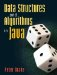# Recursion

The chapter discusses recursion, a powerful technique for designing algorithms. Section 9.1 uses a puzzle to introduce the recursive way of thinking. The analysis of recursive algorithms requires new techniques, which are explained in Section 9.2. Two recursive sorting algorithms are introduced in Sections 9.3 and 9.4. While recursive algorithms are often very concise and elegant, their efficiency can sometimes be improved by converting them into a nonrecursive form, as explained in Section 9.5.

### Thinking RecursivelyData Structures and Algorithms in Java
ISBN: 0131469142
EAN: 2147483647
Year: 2004
Pages: 216
Authors: Peter Drake